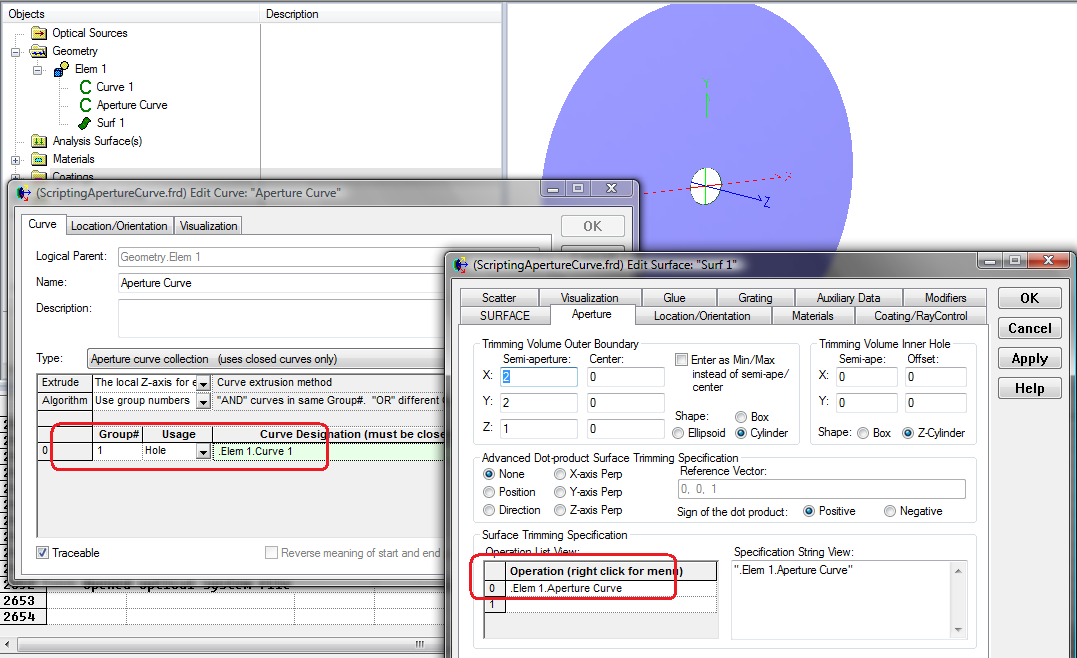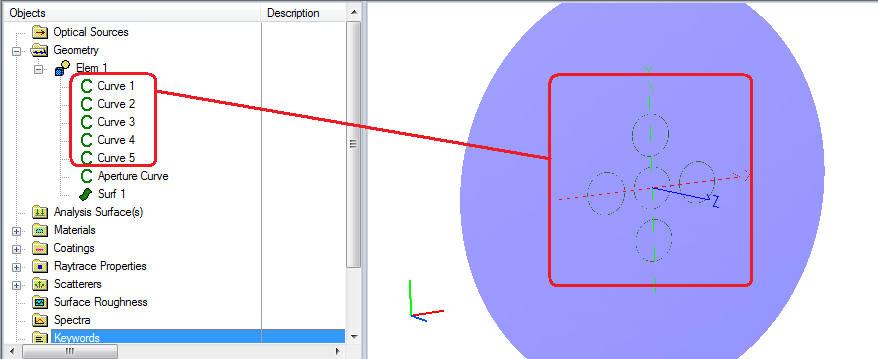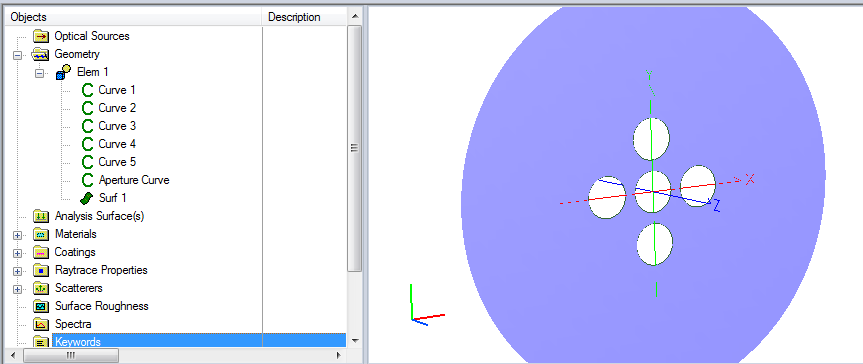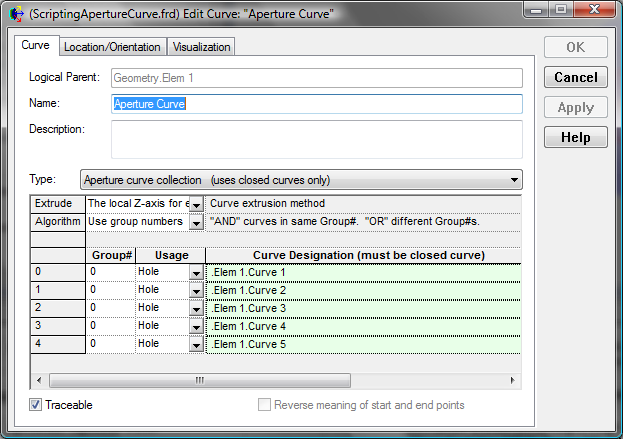# Scripting Aperture Curves

In FRED, the Aperture Curve Collection construct is used to assign an optical meaning (clear aperture, edge, obscuration, hole) to a collection of closed curves and can be used to trim a surface. A typical procedure might be:

• Create the surface.
• Create the curve describing the hole / aperture, etc.
• Create a curve of type "Aperture Curve Collection".
• Reference the original curve in the settings of the Aperture Curve.
• Reference the Aperture Curve in the Surface Trimming Specification in the surface.However, if you have a large number of these curves, it makes sense to use a script to automate the process of adding the curves into the curve list of the Aperture Curve. The example script below does this.

```Sub Main

Dim ent As T_ENTITY, i As Long
Dim apCurv As Long, curveOps() As T_APERTURECURVEOP
Dim numCurves As Long, curveOp As T_APERTURECURVEOP

apCurv = FindFullName( "Geometry.Elem 1.Aperture Curve" )
GetApertureCurve apCurv, ent, curveOps

numCurves = 0

'loop over all entities.....
For i = 0 To GetEntityCount() - 1

'for every curve that is not apCurve...
If IsCurve(i) Then
If i <> apCurv Then

'resize the array
ReDim Preserve curveOps(numCurves) As T_APERTURECURVEOP

'add an operation for this curve
curveOp.action  = "Hole"
curveOp.curv    = i
curveOp.group   = 0
curveOps(numCurves) = curveOp
numCurves = numCurves + 1

End If
End If

Next i

'update the Aperture Curve
SetApertureCurve apCurv, ent, curveOps
Update

End Sub<br>
```

The script assumes the following about the curves:

• They have already been created.
• They need to be added.
• They define holes in the surface.

Before running the scriptAfter running the scriptAssociated FRED file: ScriptingApertureCurve.frd